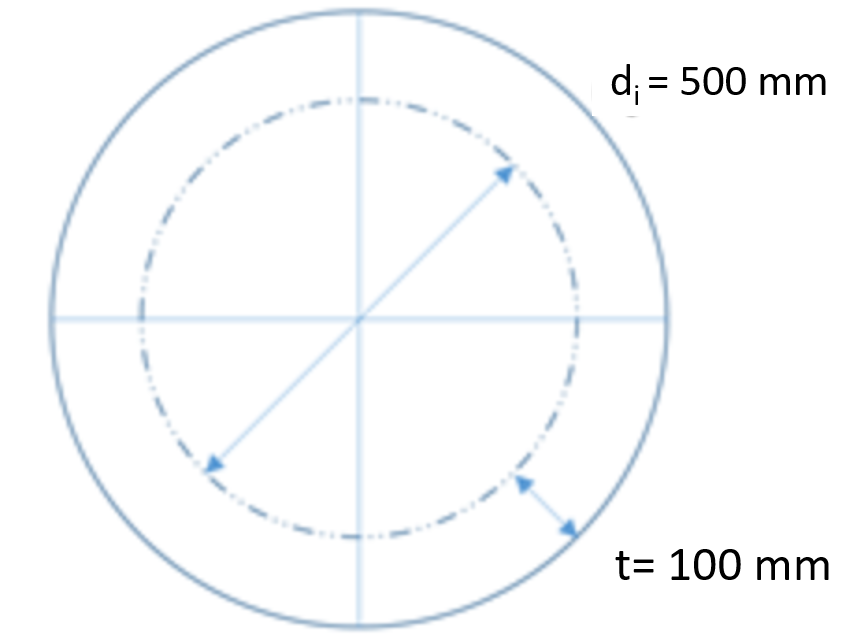## Thick Walled Vessel

Consider an air cylinder’s pressure gauge reads 1,700 kPa. If the cylinder is made of a 100 mm steel rolled plate, with an internal diameter of 500 mm, what is the tangential (hoop) stress inside the tank? Assume a thick walled cylinder.Hint
For internal pressure only, the stresses at the inside wall are:
$$\sigma_t=P_i\frac{r_o^{2}+r_i^{2}}{r_o^{2}-r_i^{2}}$$$where $$P_i$$ is the internal pressure, $$r_o$$ is the outside radius, and $$r_i$$ is the inside radius. Hint 2 $$r_o=\frac{500}{2}+100=350\:mm$$$
$$r_i=\frac{500}{2}=250\:mm$$$Because this is a thick walled vessel, the stresses caused by the internal pressure on the inside wall is: $$\sigma_t=P_i\frac{r_o^{2}+r_i^{2}}{r_o^{2}-r_i^{2}}$$$
where $$P_i$$ is the internal pressure, $$r_o$$ is the outside radius, and $$r_i$$ is the inside radius. The radii are:
$$r_o=\frac{500}{2}+100=350\:mm$$$$$r_i=\frac{500}{2}=250\:mm$$$

Solving for tangential stress:
$$\sigma_t=1,700kPa\times \frac{350^{2}+250^{2}}{350^{2}-250^{2}}$$$$$=1,700kPa\times\frac{185,000}{60,000}$$$
$$=5,242\:kPa$$\$
5,242 kPa